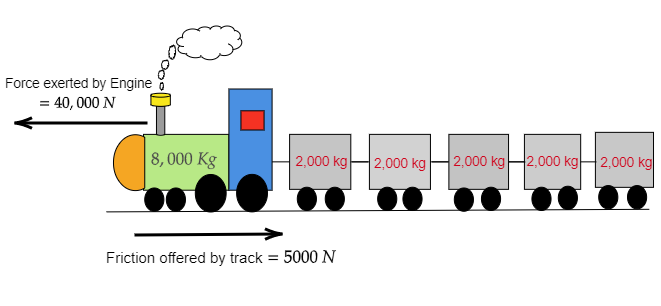# An 8000 kg engine pulls a train of 5 wagons, each of 2000 kg, along a horizontal track. If the engine exerts a force of 40000 N and the track offers a friction force of 5000 N, then calculate:$(a)$. the net accelerating force and$(b)$. the acceleration of the train.

Given: A 8000 kg engine pulls a train of 5 wagons, each of 2000 kg, along a horizontal track. If the engine exerts a force of 40000 N and the track offers a friction force of 5000 N.

To do: To calculate:

$(a)$. the net accelerating force and

$(b)$. the acceleration of the train.

Solution:$(i)$. Here given the mass of the engine $m_{engine}=8000\ kg$

Mass of each wagon $m_{wagon}=2000\ kg$

No. of wagons $=5$

Exerted force by the engine $F=40000\ N$

Friction offered by the track $f=5000\ N$

Therefore, net accelerating force $F_{net}=F-f=40,000\ N-5,000\ N=35,000\ N$

$(ii)$. Here given mass of the engine $m_{engine}=8000\ kg$

Mass of each wagon $m_{wagon}=2000\ kg$

No. of wagons $=5$

Exerted force by the engine $F=40000\ N$

Friction offered by the track $f=5000\ N$

Therefore, the net force applied $F_{net}=F-f=40000\ N-5000\ N=35000\ N$

Total mass $M_{total}=m_{engine}+m_{wagon}$

Or $M_{total}=8000\ kg+5\times2000\ kg$

Or $M_{total}=8000\ kg+10,000\ kg$

Or $M_{total}=18,000\ kg$

Therefore, acceleration $a=\frac{F_{net}}{M_{total}}$

$=\frac{35,000\ N}{18,000\ kg}$

$=1.94\ m/s^2$

Therefore, the acceleration of the train is $1.94\ m/s^2$.